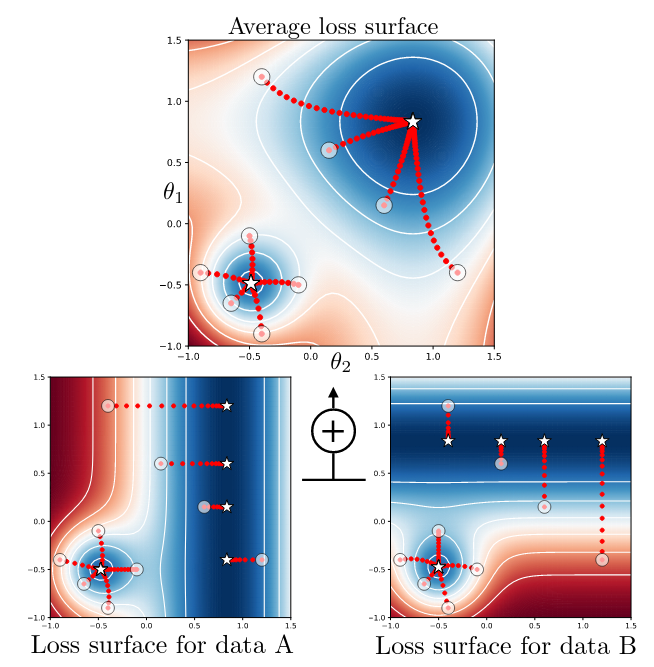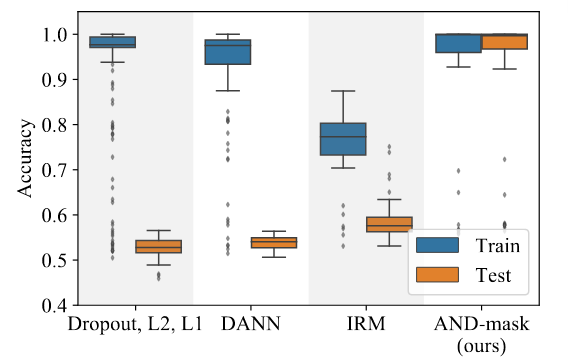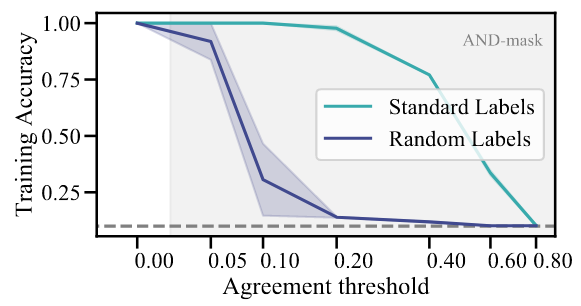# Learning explanations that are hard to vary

Posted on
deep-learning generalization

The big idea here is to use the geometric mean instead of the arithmetic mean across samples in the batch when computing the gradient for SGD. This overcomes the situation where averaging produces optima that are not actually optimal for any individual samples, as demonstrated in their toy example below:In practice, the method the authors test is not exactly the geometric mean for numerical and performance reasons, but effectively accomplishes the same thing by avoiding optima that are “inconsistent” (meaning that gradients from relatively few samples actually point in that direction).

The results are pretty impressive. Figure 6 shows the results on a difficult spiral dataset that adds a shortcut feature that is reversed in half of the dataset:Figure 8 shows how making the gradient combination more geometric (less arithmetic) makes the model unable to fit CIFAR-10 with random labels: